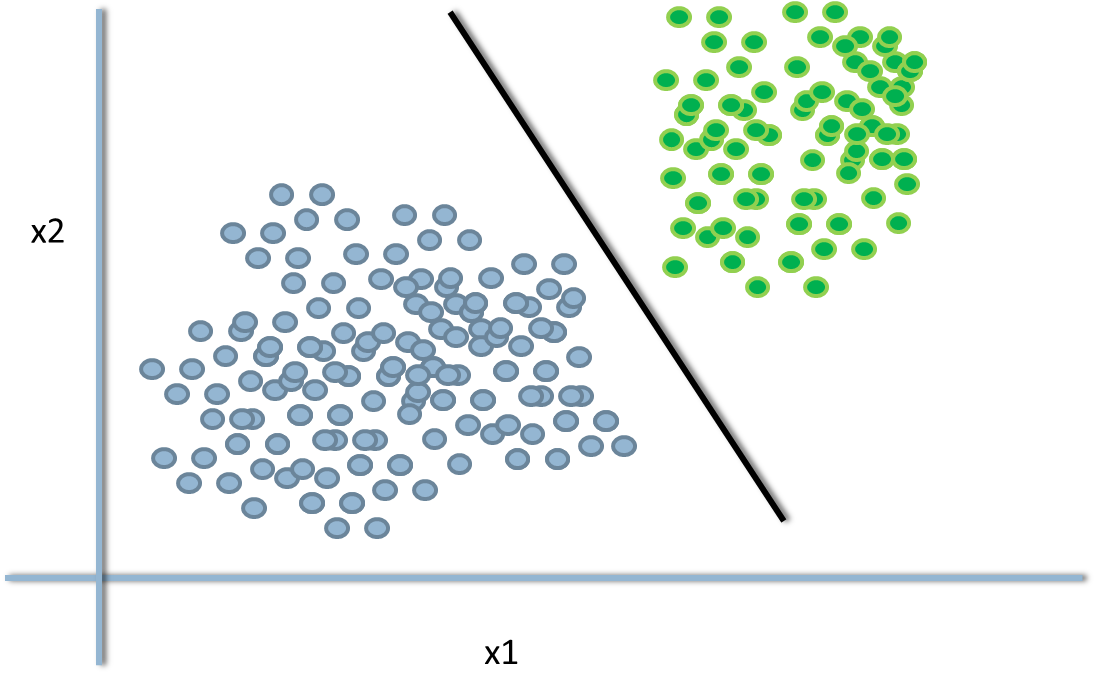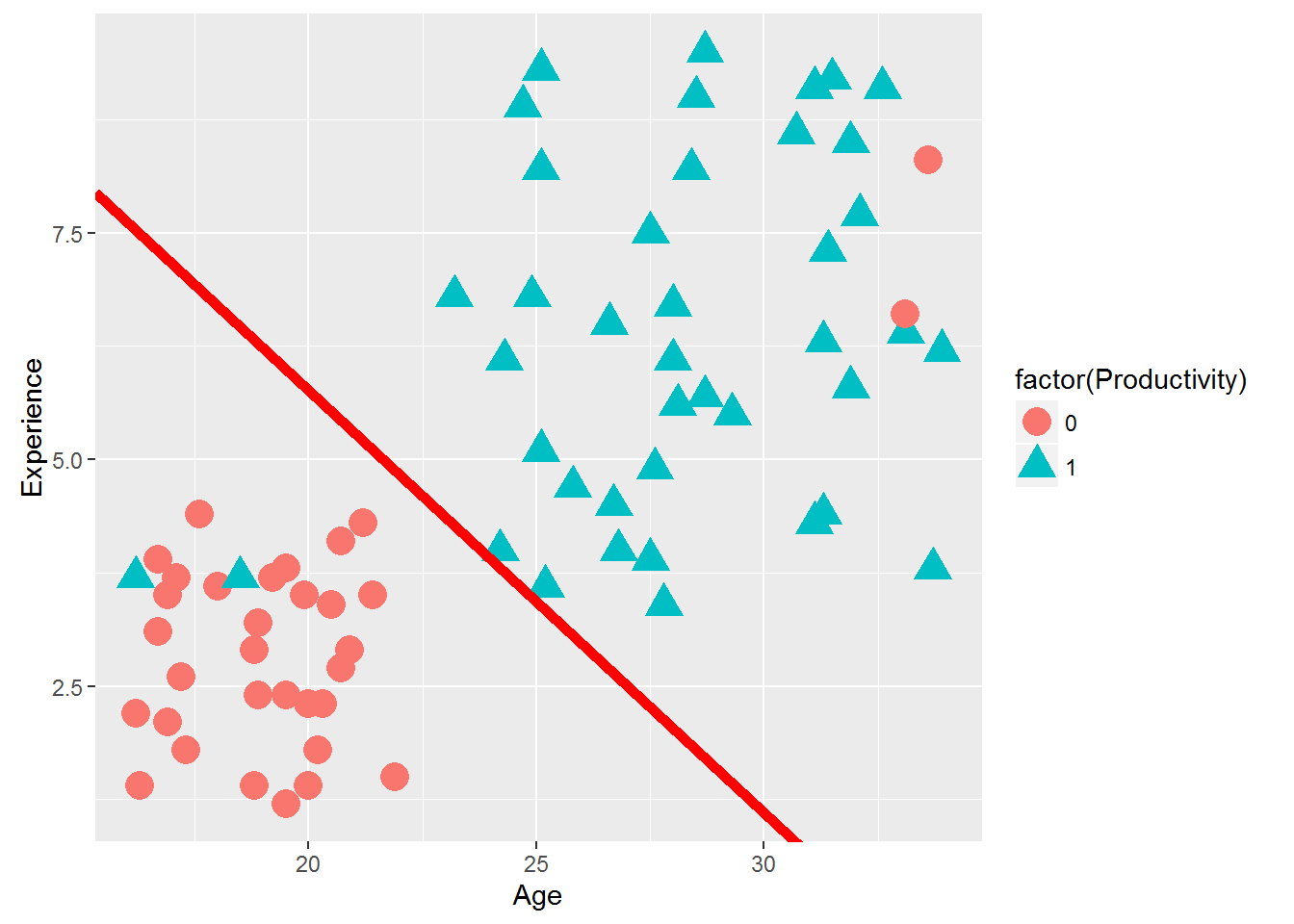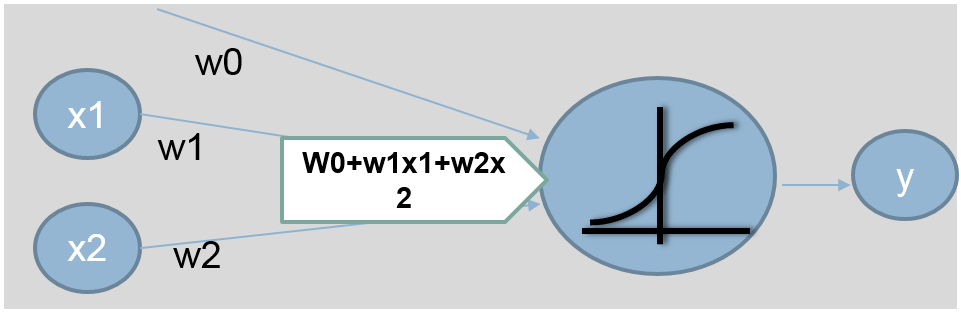• No products in the cart.

# 203.5.2 Decision Boundary – Logistic Regression

## Decision Boundary

In previous section, we studied about Neural Networks : A Recap of Logistic Regression

### Decision Boundary – Logistic Regression

• The line or margin that separates the classes
• Classification algorithms are all about finding the decision boundaries
• It need not be straight line always
• The final function of our decision boundary looks like
• Y=1 if $w^Tx+w_0>0$ ; else Y=0• In logistic regression, it can be derived from the logistic regression coefficients and the threshold.
• Imagine the logistic regression line p(y)=$e^(b_0+b_1x_1+b_2x_2)/1+exp^(b_0+b_1x_1+b_2x_2)$
• Suppose if p(y)>0.5 then class-1 or else class-0
• $log(y/1-y)=b_0+b_1x_1+b_2x_2$
• $Log(0.5/0.5)=b_0+b_1x_1+b_2x_2$
• $0=b_0+b_1x_1+b_2x_2$
• $b_0+b_1x_1+b_2x_2=0 is the line$
• Rewriting it in mx+c form
• $X_2=(-b_1/b_2)X_1+(-b_0/b_2)$
• Anything above this line is class-1, below this line is class-0
• $X_2>(-b_1/b_2)X_1+(-b_0/b_2)$ is class-1
• $X_2<(-b_1/b_2)X_1+(-b_0/b_2)$ is class-0
• $X_2=(-b_1/b_2)X_1+(-b_0/b_2)$ tie probability of 0.5
• We can change the decision boundary by changing the threshold value(here 0.5)

### LAB: Decision Boundary

• Draw a scatter plot that shows Age on X axis and Experience on Y-axis. Try to distinguish the two classes with colors or shapes (visualizing the classes)
• Build a logistic regression model to predict Productivity using age and experience
• Finally draw the decision boundary for this logistic regression model
• Create the confusion matrix
• Calculate the accuracy and error rates

### Solution

• Drawing the Decision boundary for the logistic regression model
library(ggplot2)
base<-ggplot(Emp_Productivity1)+geom_point(aes(x=Age,y=Experience,color=factor(Productivity),shape=factor(Productivity)),size=5)
base+geom_abline(intercept = intercept1 , slope = slope1, color = "red", size = 2)#Base is the scatter plot. Then we are adding the decision boundary
• Accuracy of the model1
predicted_values<-round(predict(Emp_Productivity_logit,type="response"),0)
conf_matrix<-table(predicted_values,Emp_Productivity_logit\$y)
conf_matrix
##
## predicted_values  0  1
##                0 31  2
##                1  2 39
accuracy<-(conf_matrix[1,1]+conf_matrix[2,2])/(sum(conf_matrix))
accuracy
##  0.9459459

## New Representation for Logistic Regression

$y=\frac{e^(b_0+b_1x_1+b_2x_2)}{1+e^(b_0+b_1x_1+b_2x_2)}$ $y=\frac{1}{1+e^-(b_0+b_1x_1+b_2x_2)}$ $y=g(w_0+w_1x_1+w_2x_2) where g(x)=\frac{1}{1+e^-(x)}$ $y=g(\sum w_kx_k)$#### Finding the weights in logistic regression

out(x) = $y=g(\sum w_kx_k)$

The above output is a non linear function of linear combination of inputs – A typical multiple logistic regression line

We find w to minimize $\sum_{i=1}^n [y_i – g(\sum w_kx_k)]^2$

The next post is a practice session on Non Linear Decision Boundary.

21st June 2017

### 0 responses on "203.5.2 Decision Boundary – Logistic Regression"

Statinfer Software Solutions LLP

Software Technology Parks of India,
NH16, Krishna Nagar, Benz Circle,